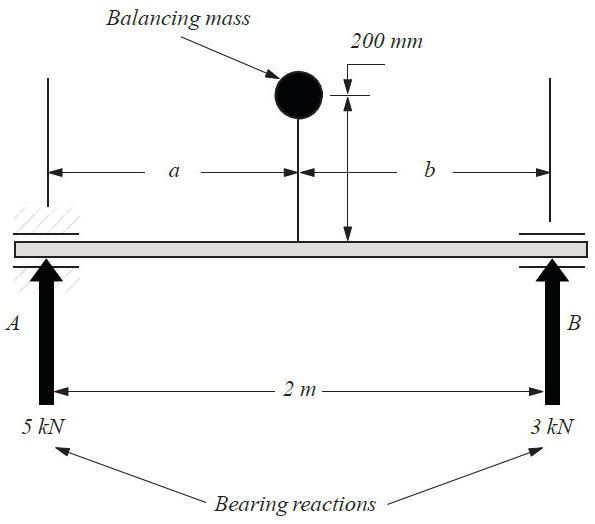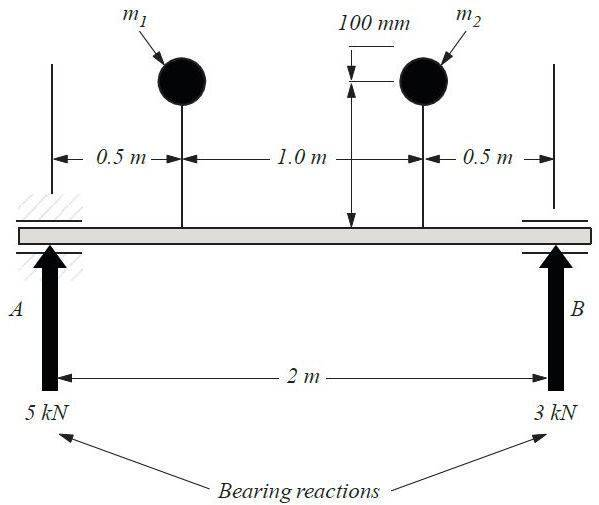# Shaft balancing

## Homework Statement

A.A shaft 2m long rotates at 1500 revs min-1 between bearings as shown. The bearings forces of 5kN and 3kN acting in the same plane as shown. A single mass is to be used to balance the shaft, so that the reactions are zero. The mass is to be placed at a radius of 200mm from the shaft centre, 180o from the direction of the bearing reactions. Determine the size and position (a and b) of the mass to be used.

B.The shaft in part A is to be balanced using two mases (m1 and m2) placed 0.5m and 1.5m from end A and 180o from the direction of the bearing reactions, each on radius arms 100mm long. Calculate the sizes of m1 and m2.

F=m*r*ω2

## The Attempt at a Solution

A.

To balance the shaft the bearing reactions must equal to centrifugal force of the mass.

RA+RB=F
F=5kN+3kN=8kN
F=m*r*ω2
8000=m*0.2*50π2
4934.8022m=8000
m=1.6211kg

RA*2-8*b=0
10-8b=0
8b=10
b=1.25m

a+b=2m
a=2-1.25=075m

B.
F1+F2=8kN

RA*2-F1*1.5-F2*0.5=0
10-1.5*F1-0.5*F2=0
1.5*F1+0.5*F2=10

F1+F2=8
1.5*F1+0.5*F2=10

Solving equation

F1=8-F2
1.5*(8-F2)+0.5*F2=10
12-1.5*F2+0.5*F2=10
F2=2kN

F1+2=8
F1=6kN

F1=m1*r*ω2
6000=m1*0.1*50π2
2467.4011m1=6000
m1=2.4317kg

F2=m2*r*ω2
2000=m2*0.1*50π2
2467.4011m2=2000
m2=0.8106kg

Did I got it right?
I'm not sure where I went wrong, but I suspect the m1 should be smaller then m2 as RA is bigger then RB. Any tips?

SammyS
Staff Emeritus
Homework Helper
Gold Member

## Homework Statement

A.
View attachment 86596
A shaft 2m long rotates at 1500 revs min-1 between bearings as shown. The bearings forces of 5kN and 3kN acting in the same plane as shown. A single mass is to be used to balance the shaft, so that the reactions are zero. The mass is to be placed at a radius of 200mm from the shaft centre, 180o from the direction of the bearing reactions. Determine the size and position (a and b) of the mass to be used.

B.
View attachment 86597

The shaft in part A is to be balanced using two mases (m1 and m2) placed 0.5m and 1.5m from end A and 180o from the direction of the bearing reactions, each on radius arms 100mm long. Calculate the sizes of m1 and m2.

F=m*r*ω2

## The Attempt at a Solution

A.

To balance the shaft the bearing reactions must equal to centrifugal force of the mass.

RA+RB=F
F=5kN+3kN=8kN
F=m*r*ω2
8000=m*0.2*50π2
4934.8022m=8000
m=1.6211kg

RA*2-8*b=0
10-8b=0
8b=10
b=1.25m

a+b=2m
a=2-1.25=075m

B.
F1+F2=8kN

RA*2-F1*1.5-F2*0.5=0
10-1.5*F1-0.5*F2=0
1.5*F1+0.5*F2=10

F1+F2=8
1.5*F1+0.5*F2=10

Solving equation

F1=8-F2
1.5*(8-F2)+0.5*F2=10
12-1.5*F2+0.5*F2=10
F2=2kN

F1+2=8
F1=6kN

F1=m1*r*ω2
6000=m1*0.1*50π2
2467.4011m1=6000
m1=2.4317kg

F2=m2*r*ω2
2000=m2*0.1*50π2
2467.4011m2=2000
m2=0.8106kg

Did I got it right?
I'm not sure where I went wrong, but I suspect the m1 should be smaller then m2 as RA is bigger then RB. Any tips?
It looks right to me.

There is an easier way to do this.

Consider: At what location along the shaft, do the bearing reaction forces produce zero torque?

•Thanks SammyS for you reply. Can you expand a bit more on that?

SammyS
Staff Emeritus
•